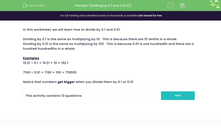# Divide by 0.1 and 0.01

In this worksheet, students will divide numbers by 0.1 and 0.01 by multiplying by 10 and 100Key stage:  KS 3

Curriculum topic:   Number

Curriculum subtopic:   Use Four Operations for All Numbers

Popular topics:   Multiplication worksheets, Division worksheets

Difficulty level:#### Worksheet Overview

In this activity, we will practise dividing by 0.1 and 0.01

Dividing by 0.1 is the same as multiplying by 10.  This is because there are 10 tenths in a whole.

Dividing by 0.01 is the same as multiplying by 100.  This is because 0.01 is one hundredth and there are a hundred hundredths in a whole.

Examples

19.21 ÷ 0.1 = 19.21 × 10 = 192.1

7,190 ÷ 0.01 = 7,190 × 100 = 719,000

Notice that numbers get bigger when you divide them by 0.1 or 0.01

Let's get started!

### What is EdPlace?

We're your National Curriculum aligned online education content provider helping each child succeed in English, maths and science from year 1 to GCSE. With an EdPlace account you’ll be able to track and measure progress, helping each child achieve their best. We build confidence and attainment by personalising each child’s learning at a level that suits them.

Get started